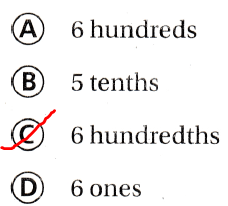# Texas Go Math Grade 4 Lesson 2.2 Answer Key Explore Decimal Place Value

Refer to our Texas Go Math Grade 4 Answer Key Pdf to score good marks in the exams. Test yourself by practicing the problems from Texas Go Math Grade 4 Lesson 2.2 Answer Key Explore Decimal Place Value.

## Texas Go Math Grade 4 Lesson 2.2 Answer Key Explore Decimal Place Value

Essential Question

How can you find the value of a digit using its place-value position?
Place value is the value of a digit according to its position in the number such as ones, tens, hundreds, and so on.
Example: 5 in 3,458 represents 5 tens, or 50

Unlock the Problem

Connect Decimals, like whole numbers, can be written in standard form, word form, and expanded form.
How can you write the value of each digit in 5.76 using decimal expander strips?

Activity Explore expanded form.

Materials: decimal expander strips

STEP 1. Place the decimal expander strip in front of you, with the largest rectangle on the left-hand side.STEP 2. Fold along the first two dashed lines as shown, keeping the largest rectangle toward the back.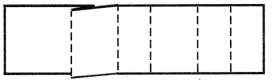STEP 3. Continue folding along the dashed lines. Then turn the paper around so that the largest rectangle is on the right-hand side.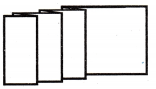STEP 4. Write 5, 7, 6, placing one digit in each section, as shown. Insert a decimal point before the 7.STEP 5. Unfold the decimal expander, and use numbers and symbols to write 5 + 0.7 + 0.06.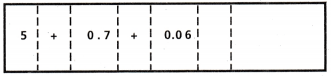STEP 6. Use the second strip of paper to make another decimal expander for 5.76. This time, write the word that names the value of each digit. The strip should read 5 ones 7 tenths 6 hundredths.Math Talk

Mathematical Processes:
Explain how you can write 3.5 as 35 tenths.
The given number is: 3.5
Now,
3.5 = 35 × 0.1
= 35 × $$\frac{1}{10}$$
= 35 tenths
Hence, from the above,
We can conclude that
We can write 3.5 as 35 tenths using the fractions and place-value positions

Example Use a place-value chart.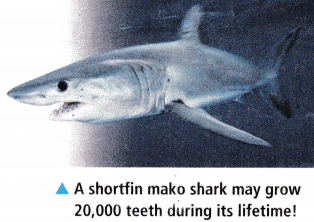Shortfin mako shark teeth range in length from 0.64 centimeters to 5.08 centimeters. Eli found a Shortfin mako shark tooth measuring 2.54 centimeters on the beach.
you can use a place-value chart to help you understand decimal place values.

Write the decimal above in the place-value chart.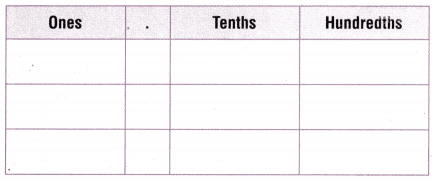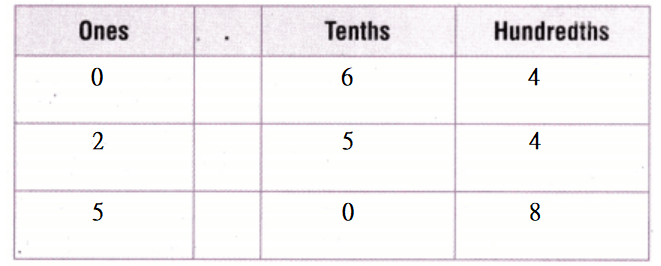What is the value of digit 4 in 2.54?
The value of the digit 4 is 4  hundredths, or 0.04.

Math Talk

Mathematical Processes:
In the whole number 277, the value of each digit is 10 times as great as the place-value position to its right. Explain why, in the decimal 2.77, the value of the digit 7 in the tenth place is 10 times as great as the value of the digit 7 in the hundredth place.
It is given that
In the whole number 277, the value of each digit is 10 times as great as the place-value position to its right
Now,
We know that,
The place-value positions for the given number is: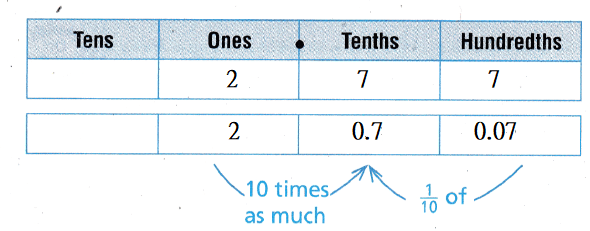Hence, from the above,
We can conclude that
In the decimal 2.77, the value of the digit 7 in the tenth place is 10 times as great as the value of the digit 7 in the hundredth place due to the rules present in the decimal number system

Share and Show

Question 1.
What is the place-value position of the digit 8 in 0.98? ____________The given number is: 0.98
Now,
The given place-value chart for 0.98 is:Now,
From the above place-value chart,
We can observe that
The digit 8 in 0.98 present in “Hundredths” place-value
Hence, from the above,
We can conclude that
The place-value position of the digit 8 in 0.98 is: 0.08

Write the value of the underlined digit.

Question 2.
2.1
The given number is: 2.1
Now,
The representation of the place-value chart for the given number is:Hence, from the above,
We can conclude that
The value of the underlined digit in the given number is: 0.1

Question 3.
0.09
The given number is: 0.09
Now,
The representation of the place-value chart for the given number is:Hence, from the above,
We can conclude that
The value of the underlined digit in the given number is: 0.09

Question 4.
6.54
The given number is: 6.54
Now,
The representation of the place-value chart for the given number is: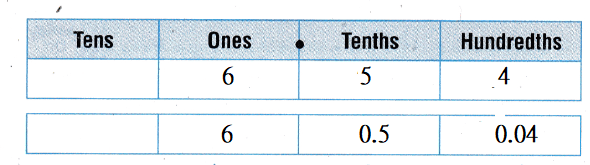Hence, from the above,
We can conclude that
The value of the underlined digit in the given number is: 0.04

Question 5.
0.3
The given number is: 0.3
Now,
The representation of the place-value chart for the given number is:Hence, from the above,
We can conclude that
The value of the underlined digit in the given number is: 0.3

Write the number in two other forms.

Question 6.
3.0 + 0.9 + 0.02
The given Expanded form is:
3.0 + 0.9 + 0.02
Now,
We know that,
The “Word Form” is a form that contains only words but not numbers
The “Expanded form” is a way to write a number by showing the value of each digit
The “Standard Form” is a form that contains only numbers but not any words
So,
The representation of the Standard form for the given expanded form is: 3.92
The representation of the Word form for the given expanded form is:
Three and Ninety-two hundredths
Hence, from the above,
We can conclude that
The representation of the Standard form for the given expanded form is: 3.92
The representation of the Word form for the given expanded form is:
Three and Ninety-two hundredths

Question 7.
seventeen hundredths
The given Word form is: Seventeen hundredths
Now,
We know that,
The “Word Form” is a form that contains only words but not numbers
The “Expanded form” is a way to write a number by showing the value of each digit
The “Standard Form” is a form that contains only numbers but not any words
So,
The representation of the Standard form for the given Word form is: 0.17
The representation of the Expanded form for the given Word form is:
0 + 0.1 + 0.07
Hence, from the above,
We can conclude that
The representation of the Standard form for the given Word form is: 0.17
The representation of the Expanded form for the given Word form is:
0 + 0.1 + 0.07

Problem Solving

Practice: Copy and Solve
Write the number in two other forms.

Question 8.
8.26
The given Standard form is: 8.26
Now,
We know that,
The “Word Form” is a form that contains only words but not numbers
The “Expanded form” is a way to write a number by showing the value of each digit
The “Standard Form” is a form that contains only numbers but not any words
So,
The representation of the Expanded form for the given Standard form is:
8 + 0.2 + 0.06
The representation of the Word form for the given Standard form is:
Eight and Twenty-six hundredths
Hence, from the above,
We can conclude that
The representation of the Expanded form for the given Standard form is:
8 + 0.2 + 0.06
The representation of the Word form for the given Standard form is:
Eight and Twenty-six hundredths

Question 9.
one and two tenths
The given Word form is:
One and two tenths
Now,
We know that,
The “Word Form” is a form that contains only words but not numbers
The “Expanded form” is a way to write a number by showing the value of each digit
The “Standard Form” is a form that contains only numbers but not any words
So,
The representation of the Expanded form for the given Word form is:
1 + 0.2
The representation of the Standard form for the given Word form is: 1.2
Hence, from the above,
We can conclude that
The representation of the Expanded form for the given Word form is:
1 + 0.2
The representation of the Standard form for the given Word form is: 1.2

Question 10.
10 + 6 + 0.7 + 0.02
The given Expanded form is:
10 + 6 + 0.7 + 0.02
Now,
We know that,
The “Word Form” is a form that contains only words but not numbers
The “Expanded form” is a way to write a number by showing the value of each digit
The “Standard Form” is a form that contains only numbers but not any words
So,
The representation of the Word form for the given Expanded form is:
Sixteen and Seventy-two hundredths
The representation of the Standard form for the given Expanded form is: 16.72
Hence, from the above,
We can conclude that
The representation of the Word form for the given Expanded form is:
Sixteen and Seventy-two hundredths
The representation of the Standard form for the given Expanded form is: 16.72

Question 12.
Explain how you write the number 7.04 in expanded form.
The given number is: 7.04
Now,
We know that,
The “Word Form” is a form that contains only words but not numbers
The “Expanded form” is a way to write a number by showing the value of each digit
The “Standard Form” is a form that contains only numbers but not any words
Now,
From the given number,
We can observe that
The given number is in Standard form
So,
The representation of the Expanded form for the given Standard form is:
7 + 0 + 0.04
Hence, from the above,
We can conclude that
The representation of the Expanded form for 7.04 is:
7 + 0 + 0.04

Use the chart for 13-14.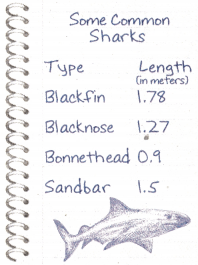Question 13.
A marine researcher recorded the lengths of some sharks he observed offshore. The length of which shark has the digit 7 in the tenths place?
It is given that
A marine researcher recorded the lengths of some sharks he observed offshore
Now,
The given chart is:Now,
The representation of the place-value table is:Now,
From the above chart,
According to the positions of the above place-value table,
We can observe that
The length of “Blackfin” has the digit 7 in the tenth place
Hence, from the above,
We can conclude that
The length of “Blackfin shark” has the digit 7 in the tenth place

Question 14.
Multi-Step What’s the Error?
Randy said that the black nose shark is one and twenty-seven tenths meters in length. Describe Randy’s error and write the decimal in word form.
It is given that
Randy said that the black nose shark is one and twenty-seven tenths meters in length
Now,
The given chart is:Now,
The representation of the place-value table is:Now,
From the above chart,
We can observe that
The length of a Blacknose Shark is: 1.27 meters
Now,
We know that,
The “Word Form” is a form that contains only words but not numbers
The “Expanded form” is a way to write a number by showing the value of each digit
The “Standard Form” is a form that contains only numbers but not any words
So,
1.27 is in the Standard form
Now,
The representation of the Word form for the given Standard form is:
One and Twenty-seven hundredths
But,
According to Randy,
The representation of the Word form for the given Standard form is:
One and Twenty-seven tenths
So,
The error is:
Since after the decimal point there are 2 numbers, the last digit must have the place-value of hundredths and after the decimal point, if there is only 1 number, then the last digit must have the place-value of a tenth
Hence, from the above,
We can conclude that
The error of Randy is:
Since after the decimal point there are 2 numbers, the last digit must have the place-value of hundredths and after the decimal point, if there is only 1 number, then the last digit must have the place-value of a tenth
The representation of the Word form for 1.27 is:
One and Twenty-seven tenths

Question 15.
H.O.T.
What’s the Question? The answer is 275 hundredths.

Question 16.
An adult kangaroo jumps 7.6 meters in a single leap. What is 7.6 written in word form?
(A) seven and one-sixth
(B) seven and six tenths
(C) seven and six hundredths
(D) seventy-six hundredths
It is given that
An adult kangaroo jumps 7.6 meters in a single leap
Now,
We know that,
The “Word Form” is a form that contains only words but not numbers
The “Expanded form” is a way to write a number by showing the value of each digit
The “Standard Form” is a form that contains only numbers but not any words
So,
The representation of 7.6 in the word form is:
Seven and Six tenths
Hence, from the above,
We can conclude that
The representation of 7.6 in the Word form is: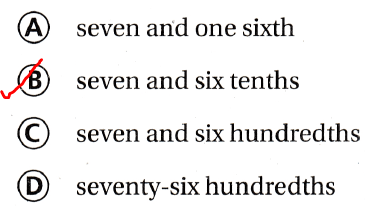Question 17.
During a blizzard, a city received 13.6 inches of snow. What is 13.6 written in expanded form?
(A) 10 + 0.3 + 0.06
(B) 10 + 3 + 0.06
(C) 10 + 3 + 0.6
(D) 10 + 3 + 6
It is given that
During a blizzard, a city received 13.6 inches of snow
Now,
We know that,
The “Word Form” is a form that contains only words but not numbers
The “Expanded form” is a way to write a number by showing the value of each digit
The “Standard Form” is a form that contains only numbers but not any words
So,
The representation of 13.6 in the Expanded form is:
10 + 3 + 0.6
Hence, from the above,
We can conclude that
The representation of 13.6 in the Expanded form is:Question 18.
A runner finished a race in 9.84 seconds. What is the value of digit 4 in 9.84?
(A) 4 hundredths
(B) 4 ones
(C) 4 tenths
(D) 4 tens
It is given that
A runner finished a race in 9.84 seconds
Now,
The representation of the place-value chart for the given number is: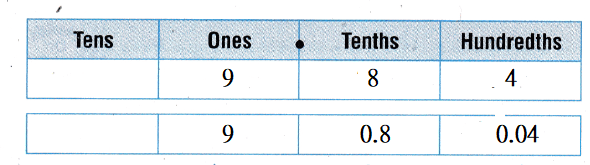Hence, from the above,
We can conclude that
The value of digit 4 in 9.84 is: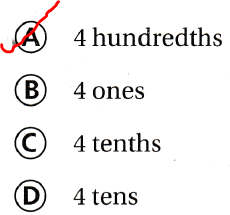TEXAS Test Prep

Question 19.
The average annual precipitation in Philadelphia, Pennsylvania, is 42.05 inches. What is the value of the digit 5 in 42.05?
(A) five tens
(B) five ones
(C) five-tenths
(D) five-hundredths
It is given that
The average annual precipitation in Philadelphia, Pennsylvania, is 42.05 inches
Now,
The representation of 42.05 in the place-value chart is:Hence, from the above,
We can conclude that
The value of digit 5 in 42.05 is: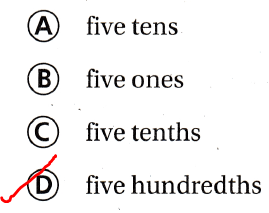### Texas Go Math Grade 4 Lesson 2.1 Homework and Practice Answer Key

Write the number in two other forms.

Question 1.
6.7
The given Standard form is: 6.7
Now,
We know that,
The “Word Form” is a form that contains only words but not numbers
The “Expanded form” is a way to write a number by showing the value of each digit
The “Standard Form” is a form that contains only numbers but not any words
So,
The representation of the Word form for the given standard form is:
Six and Seven tenths
The representation of the Expanded form for the given Standard form is:
6 + 0.7
Hence, from the above,
We can conclude that
The representation of the Word form for the given standard form is:
Six and Seven tenths
The representation of the Expanded form for the given Standard form is:
6 + 0.7

Question 2.
one and twelve hundredths
The given Word form is:
One and twelve hundredths
Now,
We know that,
The “Word Form” is a form that contains only words but not numbers
The “Expanded form” is a way to write a number by showing the value of each digit
The “Standard Form” is a form that contains only numbers but not any words
So,
The representation of the Standard form for the given Word form is: 1.12
The representation of the Expanded form for the given Word form is:
1 + 0.1 + 0.02
Hence, from the above,
We can conclude that
The representation of the Standard form for the given Word form is: 1.12
The representation of the Expanded form for the given Word form is:
1 + 0.1 + 0.02

Question 3.
80 + 7 + 0.9 + 0.03
The given Expanded form is:
80 + 7 + 0.9 + 0.03
Now,
We know that,
The “Word Form” is a form that contains only words but not numbers
The “Expanded form” is a way to write a number by showing the value of each digit
The “Standard Form” is a form that contains only numbers but not any words
So,
The representation of the Standard form for the given Expanded form is: 87.93
The representation of the Word form for the given Expanded form is:
Eighty-seven and Ninety-three hundredths
Hence, from the above,
We can conclude that
The representation of the Standard form for the given Expanded form is: 87.93
The representation of the Word form for the given Expanded form is:
Eighty-seven and Ninety-three hundredths

Question 4.
53.02
The given Standard form is: 53.02
Now,
We know that,
The “Word Form” is a form that contains only words but not numbers
The “Expanded form” is a way to write a number by showing the value of each digit
The “Standard Form” is a form that contains only numbers but not any words
So,
The representation of the Expanded form for the given Standard form is:
50 + 3 + 0 + 0.02
The representation of the Word form for the given Standard form is:
Fifty-three and Two hundredths
Hence, from the above,
We can conclude that
The representation of the Expanded form for the given Standard form is:
50 + 3 + 0 + 0.02
The representation of the Word form for the given Standard form is:
Fifty-three and Two hundredths

Write the value of the underlined digit.

Question 5.
3.6
The given number is: 3.6
Now,
The representation of the place-value chart for the given number is:Hence, from the above,
We can conclude that
The value of digit 6 in the given number is: 0.6

Question 6.
0.04
The given number is: 0.04
Now,
The representation of the place-value chart for the given number is:Hence, from the above,
We can conclude that
The value of digit 4 in the given number is: 0.04

Question 7.
9.05
The given number is: 9.05
Now,
The representation of the place-value chart for the given number is: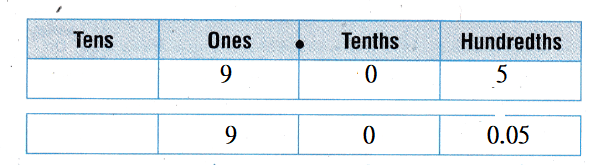Hence, from the above,
We can conclude that
The value of digit 0 in the given number is: 0

Question 8.
2.81
The given number is: 2.81
Now,
The representation of the place-value chart for the given number is: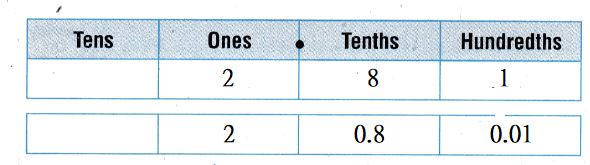Hence, from the above,
We can conclude that
The value of digit 1 in the given number is: 0.01

Problem Solving

Use the chart for 9-10.Question 9.
Jordan recorded the distances he walked this week. On which day did he walk two and twenty-five hundredths kilometers?
It is given that
Jordan recorded the distances he walked this week
Now,
The given chart is:Now,
The representation of the place-value table is:Now,
The representation of the given Word form in the standard form is: 2.25
Now,
From the above chart,
According to the place-value table,
We can observe that
On Friday,
Jordan walked 2.25 kilometers
Hence, from the above,
We can conclude that
On Friday, Jordan walked two and twenty-five hundredth kilometers

Question 10.
Leah said Jordan walked 3 and 5 tenths kilometers on Wednesday. What error did she make?
It is given that
Leah said Jordan walked 3 and 5 tenths kilometers on Wednesday
Now,
The given chart is:Now,
From the given chart,
We can observe that
Jordan walked 3.05 kilometers on Wednesday
But,
According to Leah,
Jordan walked 3 and 5 tenths kilometers on Wednesday
So,
The representation of three and five-tenths in the standard form is: 3.5
So,
The error is:
Since after the decimal point there are 2 numbers, the last digit must have the place-value of hundredths and after the decimal point, if there is only 1 number, then the last digit must have the place-value of a tenth
Hence, from the above,
We can conclude that
The error made by Leah is:
Since after the decimal point there are 2 numbers, the last digit must have the place-value of hundredths and after the decimal point, if there is only 1 number, then the last digit must have the place-value of a tenth

Lesson Check

Question 11.
What is 8.07 written in word form?
(A) eight and seven-tenths
(B) eighty-seven tenths
(C) eight and seven hundredths
(D) eighty-seven hundredths
The given Standard form is: 8.07
Now,
We know that,
The “Word Form” is a form that contains only words but not numbers
The “Expanded form” is a way to write a number by showing the value of each digit
The “Standard Form” is a form that contains only numbers but not any words
So,
The representation of 8.07 in the Word form is:
Eight and Seven hundredths
Hence, from the above,
We can conclude that
The representation of 8.07 in the Word form is:Question 12.
What is the value of the underlined digit in 37.08?
(A) 8 tenths
(B) 8 ones
(C) 8 tens
(D) 8 hundredths.
The given number is: 37.08
Now,
The representation of the given number in the place-value chart is: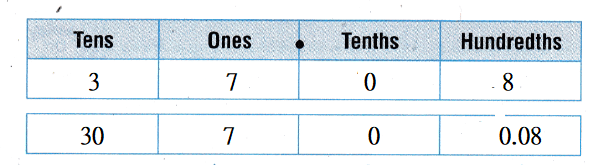Hence, from the above,
We can conclude that
The value of digit 8 in 37.08 is: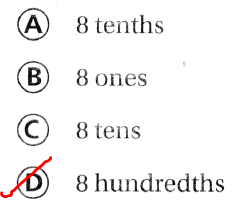Question 13.
What is the expanded form for 70.26?
(A) 7 + 2 + 0.6
(B) 70 + 2 + 0.6
(C) 7 + 0.2 + 0.06
(D) 70 + 0.2 + 0.06
The given Standard form is: 70.26
Now,
We know that,
The “Word Form” is a form that contains only words but not numbers
The “Expanded form” is a way to write a number by showing the value of each digit
The “Standard Form” is a form that contains only numbers but not any words
So,
The representation of 70.26 in the Expanded form is:
70 + 0 + 0.2 + 0.06
Hence, from the above,
We can conclude that
The Expanded form for 70.26 is:Question 14.
What is thirty-seven and nine-tenths written in expanded form?
(A) 30 + 7 + 0.9
(B) 3 + 0.7 + 0.09
(C) 30 + 7 + 0.09
(D) 37 + 0.9
The given Word form is:
Thirty-seven and nine-tenths
Now,
We know that,
The “Word Form” is a form that contains only words but not numbers
The “Expanded form” is a way to write a number by showing the value of each digit
The “Standard Form” is a form that contains only numbers but not any words
So,
The representation of the given Word form in the Expanded form is:
30 + 7 + 0.9
Hence, from the above,
We can conclude that
The representation of Thirty-seven and nine-tenths in the Expanded form is:Question 15.
What is the place value of 9 in 42.96?
(A) tenths
(B) ones
(C) tens
(D) hundredths
The given number is: 42.96
Now,
The representation of 42.96 in the place-value chart is:Hence, from the above,
We can conclude that
The place-value of 9 in 42.96 is:Question 16.
Matthias walked around the track for 9.28 minutes. What is the value of digit 2 in 9.28?
(A) 2 ones
(B) 2 tenths
(C) 2 hundred
(D) 2 hundredths
It is given that
Matthias walked around the track for 9.28 minutes
Now,
The representation of 9.28 in the place-value chart is:Hence, from the above,
We can conclude that
The value of digit 2 in 9.28 is: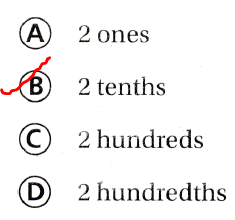Question 17.
Multi-Step During a rainstorm, Dallas received 1.25 inches of rain and Arlington received 3.25 inches. which statement is true about 1.25 and 3.25?
(A) The value of 2 is 2 tenths.
(B) The value of 1 is 1 hundred.
(C) The value of 5 is 5 tenths.
(D) The value of 2 is 2 tens.
It is given that
During a rainstorm, Dallas received 1.25 inches of rain and Arlington received 3.25 inches.
Now,
The representation of 1.25 in the place-value chart is: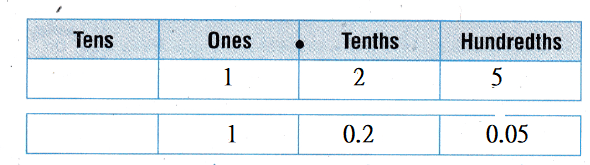Now,
The representation of 3.25 in the place-value chart is:Hence, from the above,
We can conclude that
The statement that is true about 1.25 and 3.25 is: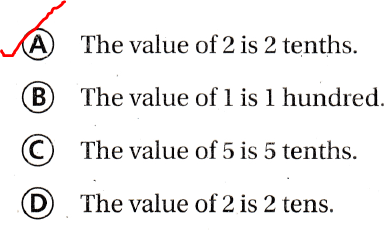Question 18.
Multi-Step What is the value of the digit to the right of the underlined digit in 76.56?
(A) 6 hundred
(B) 5 tenths
(C) 6 hundredths
(D) 6 ones